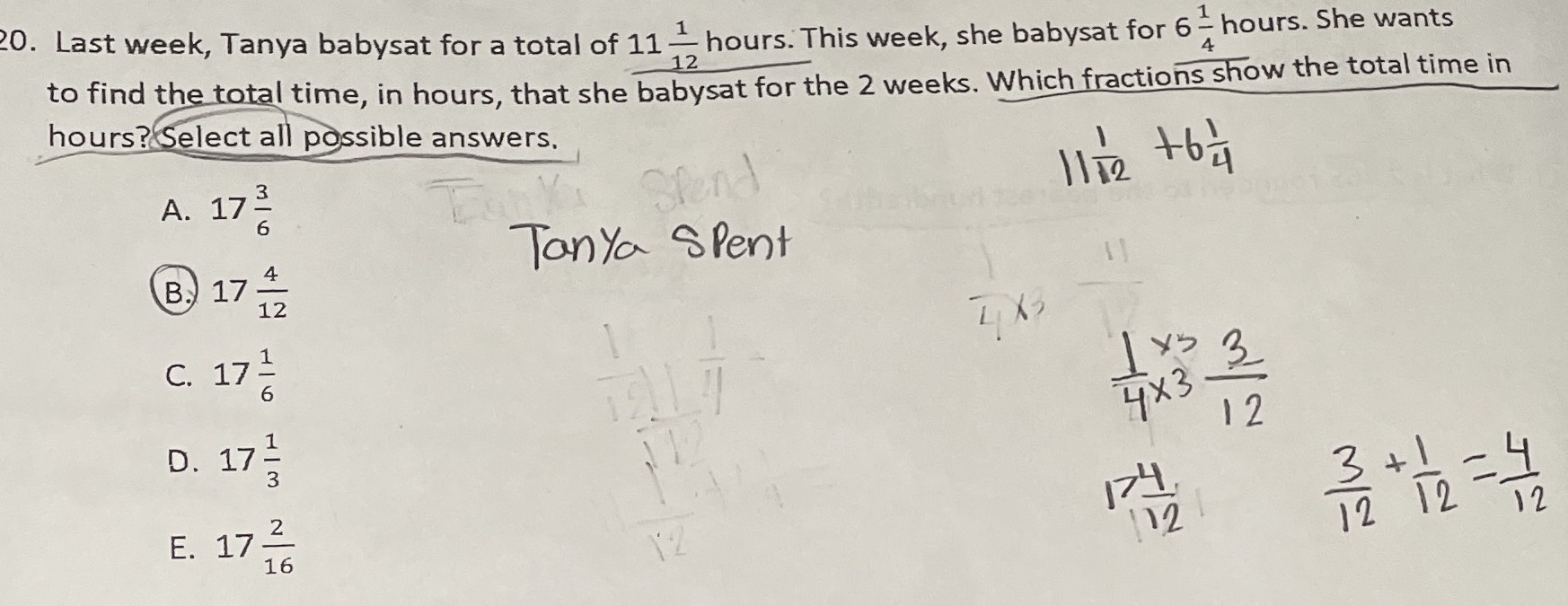### ¿Todavía tienes preguntas de matemáticas?

Pregunte a nuestros tutores expertos
Algebra
Pregunta20. Last week, Tanya babysat for a total of $$11 \frac { 1 } { 12 }$$ hours. This week, she babysat for $$6 \frac { 1 } { 4 }$$ hours. She wants to find the total time, in hours, that she babysat for the $$2$$ weeks. Which fractions show the total time in hours? Select all possible answers.

A. $$17 \frac { 3 } { 6 }$$

B. $$17 \frac { 4 } { 12 }$$

C. $$17 \frac { 1 } { 6 }$$

D. $$17 \frac { 1 } { 3 }$$

E. $$17 \frac { 2 } { 16 }$$